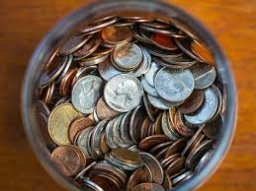# Probability 81395

What is the probability that heads come up 5 times a row when tossing a coin? Write the result as a fraction.

p =  1/32

### Step-by-step explanation:Did you find an error or inaccuracy? Feel free to write us. Thank you!

Tips for related online calculators
Need help calculating sum, simplifying, or multiplying fractions? Try our fraction calculator.
Would you like to compute the count of combinations?